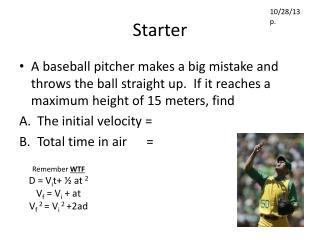DownloadDownload PresentationStarter

# Starter

Télécharger la présentation## Starter

- - - - - - - - - - - - - - - - - - - - - - - - - - - E N D - - - - - - - - - - - - - - - - - - - - - - - - - - -
##### Presentation Transcript

1. 10/28/13 p. Starter • A baseball pitcher makes a big mistake and throws the ball straight up. If it reaches a maximum height of 15 meters, find • The initial velocity = • Total time in air = Remember WTF D = Vit+ ½ at 2 Vf = Vi + at Vf 2 = Vi 2 +2ad

2. Eureka

3. Newton’s 2nd Law Force = Mass x acceleration Newton = kilogram x meter/second 2 (remember 1000 grams = 1 kilogram) & Weight is a force…. So Weight = mass x gravity

4. Practice Problems • A man hits a golf ball (0.2 kg) which accelerates at a rate of 20 m/s2. • What amount of force acted on the ball?

5. Practice Problems • You give a shopping cart a shove down the aisle. The cart is full of groceries and has a mass of 18 kg. The cart accelerates at a rate of 3 m/s2. • How much force did you exert on the cart?

6. Practice Problems • The wind pushes a paper cup along the sand at a beach. The cup has a mass of 25 grams ( = ? kg) and accelerates at a rate of 5 m/s2. • How much force (in Newtons) is the wind exerting on the cup?

7. Practice Problem • How much force would it take to push a friend on the swing who has a mass of 70kg to accelerate at the rate of 4 m/s2?

8. Practice Problem • A worker drops his hammer off the roof of a house. The hammer has a mass of 9kg, and gravity accelerates it at the usual 9.8 m/s2. • How much force does the earth apply to the hammer?

9. Practice Problem • A block of ice with a mass of 10 kg is resting on a frozen pond. • How much force will it take to accelerate the ice at 0.25 m/ sec2.

10. Practice Problem • A force of 15 Newtons is applied to an object with a mass of 5 kilograms. • What is the acceleration? • Determine the object's final velocity after 10 seconds??

11. Practice Problem • A car whose mass is 1000 kg is traveling at a constant speed of 10 m/s. Neglecting any friction, how much force will the engine have to supply to keep going the same speed? *tricky question, think INERTIA, look at the units

12. Closure • Write a story problem using Newton’s second law. Solve and show your work.